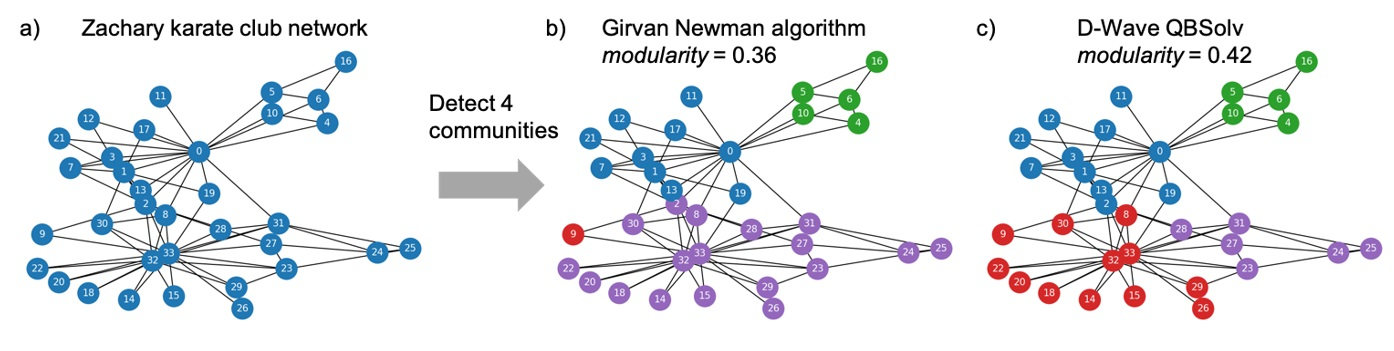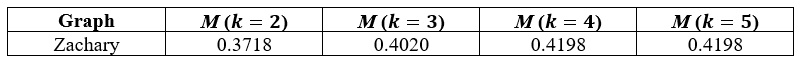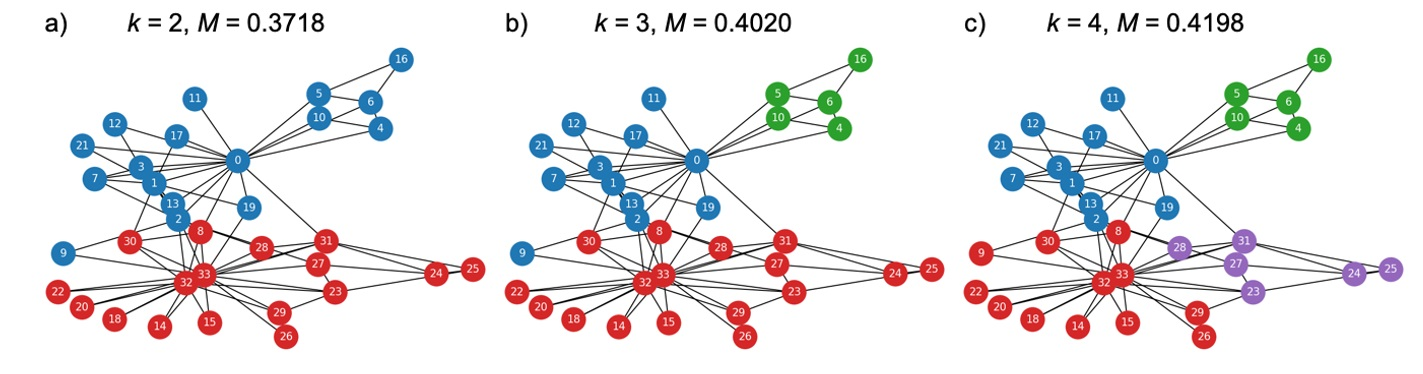# Community Detection using Hybrid Quantum Annealing on Amazon Braket – Part 1

by Jason Zhu, Kyle Brubaker, and Martin Schuetz | on | in | Permalink |  Share

As of 11/17/2022, D-Wave is no longer available on Amazon Braket and has transitioned to the AWS Marketplace. Therefore, information on this page may be outdated. Learn more.

Many customers are facing the challenge of efficiently extracting information hidden within complex network structures. For example, a healthcare insurance company needs to identify fraudulent claims through detecting abnormal relationships between patients and providers, a finance company needs an anti-money laundering tool that can detect abnormal transactions between different entities, or a marketing company needs to segment audience for targeted marketing campaigns. All such problems are related to extracting network entity relationships and are known as community detection problems.

Approaches to solving community detection problems have been evolving rapidly over the past decade, from greedy algorithms such as Girvan-Newman  and Louvain , to nature-inspired algorithms like extremal optimization , and to deep learning models . Recently Negre et. al.  explored and demonstrated the ability of a quantum computer, in particular a quantum annealer, to solve community detection as an optimization problem.

This post is the first of a two-part series about solving community detection using a hybrid classical-quantum annealing algorithm on Amazon Braket:

• In this post, part 1, we provide a step-by-step guide on how to formulate community detection as a Quadratic Unconstrained Binary Optimization (QUBO) problem, similar to the work done by Negre et. al. . We then demonstrate how to use the open source QBSolv library, which provides quantum-classical hybrid solvers for QUBO problems, using a combination of classical compute resources and D-Wave quantum annealers, to solve community detection problems on Amazon Braket.
• In Part 2, we will apply this quantum annealing-based community detection method to real-world networks and provide a systematic study about the solution performance and its scalability.

The detailed code implementation and tutorial notebook of using QBSolv for community detection in complex networks can be found in our AWS GitHub code repository.

## Modularity-based Community Detection

The general notion of community structure in complex networks was first proposed and analyzed by Girvan and Newman . The basic idea is to divide a network (or graph) into sets of nodes belonging to different communities (also called clusters), where nodes within any of these communities are highly connected (high intra-connectivity), while nodes in different communities are less connected (low inter-connectivity).

To assess the quality of a particular network division into communities, Newman and Girvan introduced a metric called modularity M. The modularity metric compares the connectivity of edges within communities to the connectivity of a network (the so-called null model) where edges would be randomly placed under the constraint that the expected degree of each node matches the degree of the node in the original graph .

Formally, we consider a graph G with corresponding adjacency matrix Aij describing the weight between nodes i and j. For the null model, the expected number of edges between nodes i and j is (approximately) given by gigj/2m, where gi = ∑j Aij is the degree of node i  and m=1/2∑i gi is the total number of weights in the graph. Taking a null model as the baseline , one can then define the modularity M as the difference between the actual weight Aij and the expected weight within a null model gigj/2m, i.e., Bij = Aij – gigj/2m, summed over all pairs of vertices i, j that fall within the same group.

Introducing a conventional pre-factor for normalization, we then arrive at the modularity M defined aswhere the Kronecker-delta δ(ci , cj ) is 1 if node i and node j are in the same community and 0 otherwise. The goal is to maximize the modularity M through optimizing community assignments ci for every node i in the graph. This problem is known to be NP-hard .

## Community Detection as a QUBO Problem

To solve the community detection problem, many heuristic search algorithms have been developed . In this post, we focus on formulating the community detection problem as a Quadratic Unconstrained Binary Optimization (QUBO) problem, and then demonstrate how to use D-Wave’s QBSolv solver on Amazon Braket to detect two or more communities in a given network.

Two communities (k = 2): Let us first consider the case where we look for a graph partitioning into k = 2 communities. In this case, we can use binary spin variables si ∈ {-1, 1} to encode which community node i belongs to. Using the fact that the term (1 + sisj)/2 yields 1 if nodes i and j belong to the same community, and 0 otherwise, the modularity metric can be expressed in compact form as :where, for convenience, the modularity matrix B has been introduced as:Using the conversion si = 2xi – 1 between spin variables si ∈ {-1, 1} and bit variables xi ∈ {0, 1}, and the fact that ∑i,jBi,j = 0, the maximization of the modularity (Eq. (3)) can be expressed equivalently as a minimization problem of QUBO form, with QUBO Hamiltonian H = -(1/m)xTBx, and with QUBO matrix Q = –B/m. QUBO matrices like this one can be readily passed to a quantum-annealing device such as D-Wave which will then try to find the optimal bit-string x which encodes the solution to our optimization problem.

Multiple communities (k > 2): Let us now turn to the more general problem of community detection with k > 2 communities. In order to formulate the k-community detection problem in canonical QUBO form (as required for quantum-native and quantum-inspired annealing), we first one-hot encode the binary variables xi, and then construct the QUBO Hamiltonian.

We use a one-hot-encoding scheme where we set xi, c = 1 if node i belongs to community c, and xi, c = 0 otherwise, i.e.,With this encoding we need k variables per logical node, and the size of the binary decision vector x increases from a vector of length N for the two-community use case to k × N for the k-community use case. Specifically, we set x = ( x1,1 , x2,1 , … , xN,1 , … , x1,k , x2,k , … , xN,k ).

We formulate the k > 2 community problem as a binary minimization problem, and we can then construct the k-community QUBO Hamiltonian with HM = -(1/m)kc=1xcTBxc in which every term in the sum describes a binary community detection problem for a given community c.

Introducing the generalized modularity matrix Β of size kN × kN and block-diagonal form with B along the diagonal as shown in Eq. (5), we can re-write the k-community detection problem as a binary minimization problem within the canonical QUBO format (where the original multiclass variables are embedded into a larger number of binary variables):Since each node i = 1, … , N must be in exactly one community c = 1, … , k, we need to add a penalty term to constrain the solution for community assignment. Formally, this constraint can be written as: kc=1 xi,c = 1 with i = 1, … , N.

This linear constraint can be added to our QUBO problem as a quadratic penalty term:with positive pre-factor P > 0 enforcing the constraints. To formulate the penalty term in Eq. (6) into a QUBO Hamiltonian HP = xT QP x, we re-number the binary decision vector using a single subscript from 1 to kN, that is x = ( x1,1 , x2,1 , … , xN,1 , … , xN,k ) = ( x1 , x2 , … , xN , … , xkN ). The penalty term Eq. (6) can then be rewritten as:where b is a vector of all ones and V = [IN, … , IN] is a highly structured matrix of size N × kN with N × N identity matrices stacked horizontally next to each other. If the weight P is large enough, for example setting P in a range of 1/N to 10/N, the penalty Hamiltonian HP will drive the solution towards one in which all constraints are satisfied, with Vx = b. Since the Hamiltonian HP is quadratic, up to an irrelevant constant it can be rewritten in canonical QUBO form as HP = xT QP x, with QP = P(VTV – 2 diag(VTb)).

Finally, combining the modularity objective in Eq. (5) and the penalty Hamiltonian in Eq. (7), we arrive at the final QUBO Hamiltonian for the general community detection with k classes:## Solving Network Community Detection on Amazon Braket

After bringing the community detection problem into a QUBO form (Eq. (8)), we are going to solve it using the QBSolv open-source library on Amazon Braket.

QBSolv is a hybrid solver that decomposes large QUBO problems into smaller QUBO sub-problems. The sub-problems are then solved individually via either D-Wave QPUs or a classical Tabu search solver. The solution to the original QUBO problem is then constructed by stitching together the results of the smaller sub-problems. More technical details can be found in the D-Wave QBSolv whitepaper and its GitHub code repository. Hereafter, we refer the QBSolv solver uses D-Wave QPUs as hybrid solver, and the one uses Tabu search alone as classical solver.

With Amazon Braket we can easily switch back and forth between the classical and hybrid solution strategies. The following code example shows how to execute the QBSolv classical solver and the QBSolv hybrid solver on Amazon Braket:

``````import minorminer
import networkx as nx
from dwave_qbsolv import QBSolv
from dwave.system.composites import FixedEmbeddingComposite
from qbsolv_community import create_qubo_dict

my_bucket = "amazon-braket-Your-Bucket-Name" # the name of the S3 braket bucket
my_prefix = "Your-Folder-Name" # the name of the folder in the bucket
s3_folder = (my_bucket, my_prefix)

qubo_mx = create_qubo_dict(graph, k) # custom function for Eq.8

# set solver parameters
solver_limit = 100
num_repeats = 1
seed = 1

# execute optimization task using QBSolv classical solver
response_classical = QBSolv().sample_qubo(qubo_mx, num_repeats=num_repeats, solver_limit=solver_limit, seed=seed)

# call QBSolv hybrid classical/quantum solver on D-wave advantage

# find embedding of subproblem-sized complete graph to the QPU
G_sub = nx.complete_graph(solver_limit)
embedding = minorminer.find_embedding(G_sub.edges, system.edgelist)

# use the FixedEmbeddingComposite() method with a fixed embedding
solver = FixedEmbeddingComposite(system, embedding)

# execute optimization task using QBSolv hybrid solver
``````

As shown in the code above, there are three key hyperparameters for QBSolv: `solver_limit` is the maximum number of variables for sub-QUBOs which is constrained by qubits of the hardware and the overhead of embedding between source graph and D-Wave QPU topology; `num_reads`, aka number of shots, refers to the number of times the annealing process is performed; and `num_repeats` is the maximum iterations to repeat QBSolv solver execution to discover a new best solution. For community detection problems, we recommend to start with the default hyperparameter values listed in the precedent code example and then do grid search to find optimal ones that would work the best for a specific graph.

### Results of Community Detection using D-Wave QBSolv on Amazon Braket

We illustrate community detection in Figure 1 using a well-known social network called Zachary’s karate club. As shown in Figure 1 (b, c), even for small graphs like Zachary’s karate club, the community assignment from QBSolv (Figure 1(c)) is better than the result found by the Girvan Newman algorithm (Figure 1(b)), as implemented within the NetworkX library for community detection.Figure 1: a) Zachary’s karate club network without community assignment; b) Solution with 4 communities using the Girvan Newman algorithm yields a modularity value M = 0.36; c) The D-Wave QBSolv classical solver and hybrid solver both find a solution with higher modularity with M = 0.42.

The number of communities k is a hyperparameter and needs to be set before constructing the QUBO matrix in Eq. (8). For example, the Zachary graph has N = 34 nodes. When searching for an assignment with k = 4 communities, the QUBO matrix will have a size of kN × kN = 136 × 136, because of the overhead of breaking down a multi-class problem into the QUBO framework with binary variables. For networks without prior information of the number of communities, we need to scan different k values via different QBSolv jobs and choose an optimal k with the highest modularity value. For networks with a pre-defined k, we can directly set k to the desired value and use QBSolv to find a solution for community assignment.

In Table 1, we show how to find the optimal number of communities for the Zachary’s karate club network by scanning k values and find the k value with the highest modularity value. In this case, the solution with 4 communities achieves the highest modularity value with M = 0.4198. When setting k = 5 or higher, QBSolv was found to still return a solution with a 4-community assignment because k = 4 is the optimal solution for the Zachary network.Table 1: Modularity values (M) of the Zachary’s karate club network by setting the number of communities to k = 2, 3, 4, and 5 as a hyperparameter in QBSolv solvers. Note that with setting k = 5, QBSolv still returned a solution with a 4-community assignment because k = 4 is the optimal solution for the Zachary network.

In Figure 2, we visualize the community structure for the Zachary graph with k = 2, 3, and 4 communities.Figure 2: Partitions of the Zachary’s karate club network with different number of communities k and the corresponding modularity values M as found by the D-Wave QBSolv solver.

## Conclusion

In this post, we showed how to use quantum annealing to solve community detection problems, which have applications in areas like social network analysis, healthcare network analysis, and collusive fraud detection. We formulated community detection problems as QUBO problems. We then solved the resulting QUBO problems using both the QBSolv classical solver and hybrid solver with the D-Wave quantum annealing device Advantage 4.1 on Amazon Braket. Using this approach, the quality of community structure detected in the sample Zachary karate club network, as quantified by the modularity metric, was found to surpass the solution quality from heuristic methods such as the Girvan Newman algorithm.

In Part 2 of this post series, we will demonstrate community detection in broad real-world networks  and compare results to published work .  You can run the experiments in this blog post by following our tutorial notebook. The notebook also contains code examples for community detection in real-world networks and synthetic networks.

### References:

1. Girvan, M. and Newman, M. E. J (2002) Community structure in social and biological networks. PNAS, 99 (12) 7821-7826
1. Traag, V.A., Waltman, L. & van Eck, N.J. From Louvain to Leiden (2019) Guaranteeing well-connected communities. Sci Rep 9, 5233
1. Duch, Jordi and Arenas, Alex (2005) Community detection in complex networks using extremal optimization. Phys. Rev. E 72, 027104
1. Liu, Fanzhen and Xue, Shan et. al. (2020) Deep Learning for Community Detection: Progress, Challenges and Opportunities. IJCAI, 4981 – 4987
1. Negre, CFA and Ushijima-Mwesigwa H, Mniszewski SM (2020) Detecting multiple communities using quantum annealing on the D-Wave system. PLOS ONE 15(2): e0227538
1. Newman, M. E. J. and Girvan, M. (2004) Finding and evaluating community structure in networks. Phys. Rev. E 69, 026113
1. Fortunato, Santo and Hric, Darko. (2016) Community detection in networks: A user guide. Physics Reports 09, 002
1. Sobolevsky, Stanislav and Campari, Riccardo and Belyi, Alexander and Ratti, Carlo. (2014) General optimization technique for high-quality community detection in complex networks. Phys. Rev. E 90, 012811
1. Rossi, Ryan A. and Ahmed, Nesreen K. (2015) The Network Data Repository with Interactive Graph Analytics and Visualization. AAAI https://networkrepository.com